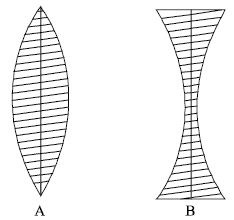Physics

# Lens and their ClassificationA lens is a transparent refractive medium bounded by two spherical surfaces. Lenses are of two types:

i) Convex lens or converging lens

ii) Concave lens or diverging lens

Convex lens: The lens whose mid portion is thick but the edges are thin is called convex lens. As the convex lens can converge a beam of parallel light rays after refraction hence it is also called converging lens. [ Fig. A]Concave lens: The lens whose mid portion is thin but the edges are thick is called concave lens. As the concave lens can diverge a beam of parallel light rays after refraction hence it is also called diverging lens. [Fig. B]